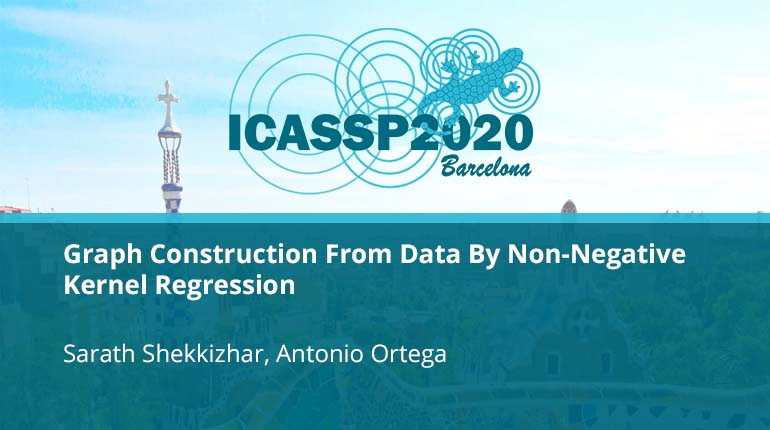# Graph Construction From Data By Non-Negative Kernel Regression

Collection:Data driven graph constructions are often used in machine learning applications. However, learning an optimal graph from data is still a challenging task. $K$-nearest neighbor and $\epsilon$-neighborhood methods are among the most common graph constructio
• IEEE MemberUS $11.00 • Society MemberUS$0.00
• IEEE Student MemberUS $11.00 • Non-IEEE MemberUS$15.00
Purchase

## Graph Construction From Data By Non-Negative Kernel Regression

Data driven graph constructions are often used in machine learning applications. However, learning an optimal graph from data is still a challenging task. $K$-nearest neighbor and $epsilon$-neighborhood methods are among the most common graph constructio